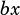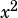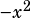(4x + 4)(ax – 1) – x^2 + 4

In the expression above, a is a constant. If the expression is equivalent to bx, where b is a constant, what is the value of b?
A) -5
B) -3
C) 0
D) 12

No idea how to solve this! I tried factoring this way and that, expanding, setting everthing on left in form of x (….) + 4 = bx, but no go. Help!

The trick is to recognize that if the expression simplifies to, then that means theand constant terms must all cancel out. Because there’s aoutside the, we know thatmust result in a positiveto cancel it out. So let’s see what happens when we plug infor a.There you have it. If the whole expression simplifies to only an x term, then a must be. Plugging that in for a leaves you with –3 for b.